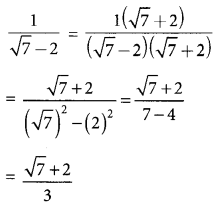## JAC Class 9 Maths Solutions Chapter 3 Coordinate Geometry Ex 3.3

Jharkhand Board JAC Class 9 Maths Solutions Chapter 3 Coordinate Geometry Ex 3.3 Textbook Exercise Questions and Answers.

## JAC Board Class 9th Maths Solutions Chapter 3 Coordinate Geometry Ex 3.3

Page-65

Question 1.
In which quadrant or on which axis do each of the points (-2, 4), (3, -1), (-1, 0), (1,2) and (-3, -5) lie? Verify your answer by locating them on the Cartesian plane.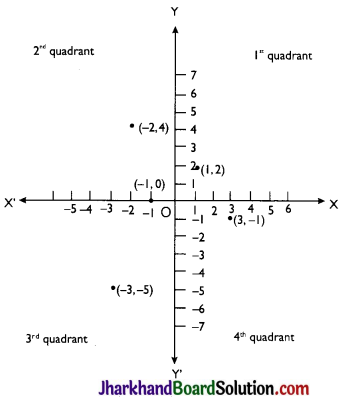(-2, 4) → Second quadrant
(3,-1) → Fourth quadrant
(-1,0) → x-axis
(1,2) → First quadrant
(-3, -5)→ Third quadrantQuestion 2.
Plot the points (x, y) given in the following table on the plane, choosing suitable units of distance on the axes.

 x -2 -1 0 1 3 y 8 7 -1.25 3 -1

Ans. Points (x, y) on the plane, 1 unit = 1 cm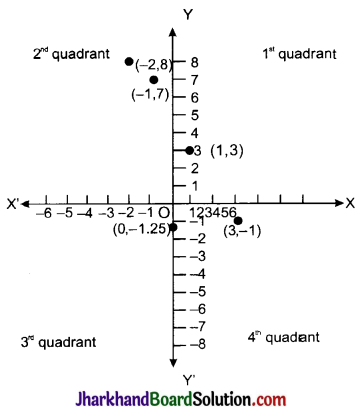## JAC Class 9 Maths Solutions Chapter 3 Coordinate Geometry Ex 3.2

Jharkhand Board JAC Class 9 Maths Solutions Chapter 3 Coordinate Geometry Ex 3.2 Textbook Exercise Questions and Answers.

## JAC Board Class 9th Maths Solutions Chapter 3 Coordinate Geometry Ex 3.2

Page – 61

Question 1.
Write the answer of each of the following questions:
(i) What is the name of horizontal and the vertical lines drawn to determine the position of any point in the Cartesian plane?
(ii) What is the name of each part of the plane formed by these two lines?
(iii) Write the name of the point where these two lines intersect.
(i) The names of horizontal line and vertical line drawn to determine the position of any point in the Cartesian plane are x-axis and y-axis respectively.
(ii) The name of each part of the plane formed by these two lines x-axis and y-axis is quadrant.
(iii) The point where these two lines intersect is called origin.Question 2.
See Fig., and write the following: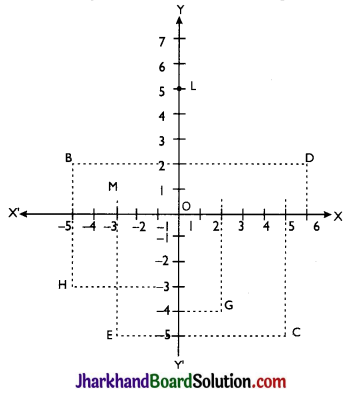(i) The coordinates of B.
(ii) The coordinates of C.
(iii) The point identified by the coordinates (-3, -5).
(iv) The point identified by the coordinates (2, -4).
(v) The abscissa of the point D.
(vi) The ordinate of the point H.
(vii) The coordinates of the point L.
(viii)The coordinates of the point M.
(i) The coordinates of B are (-5, 2).
(ii) The coordinates of C are (5, -5).
(iii) The point identified by the coordinates (-3, -5) is E.
(iv) The point identified by the coordinates (2,-4) is G.
(v) Abscissa means x-coordinate of a point. So, abscissa of the point D is 6.
(vi) Ordinate means y-coordinate of a point. So, ordinate of point H is -3.
(vii) The coordinates of the point L are (0, 5).
(viii) The coordinates of the point M are (-3,0).

## JAC Class 9 Maths Solutions Chapter 2 Polynomials Ex 2.5

Jharkhand Board JAC Class 9 Maths Solutions Chapter 2 Polynomials Ex 2.5 Textbook Exercise Questions and Answers.

## JAC Board Class 9th Maths Solutions Chapter 2 Polynomials Ex 2.5

Question 1.
Use suitable identities to find the following products:
(i) (x + 4) (x + 10)
(ii) (x + 8) (x – 10)
(iii) (3x + 4) (3x – 5)
(iv) (y2 + 3/2) (y2 – 3/2)
(v) (3 – 2x) (3 + 2x)
(i) Using identity, (x + a) (x + b)
= x2 + (a + b) x + ab
In (x + 4) (x + 10), a = 4 and b = 10
Now,
(x + 4) (x + 10)
= x2 + (4 + 10)x + (4 × 10)
= x2 + 14x+ 40

(ii) (x + 8) (x – 10)
Using identity, (x + a) (x + b)
= x2 + (a + b) x + ab
Here, a = 8 and b = -10
(x + 8) (x – 10)
= x2 + {8 + (- 10)}x + {8 × (- 10)}
= x2 + (8 – 10)x – 80
= x2 – 2x – 80

(iii) (3x + 4) (3x – 5)
Using identity, (x + a) (x + b)
= x2 + (a + b) x + ab
Here, x as 3x, a = 4 and b = – 5
(3x + 4) (3x – 5)
= (3x)2 + {4 + (-5)}3x + {4 × (-5)}
= 9x2 + 3x(4 – 5) – 20
= 9x2 – 3x – 20

(iv) (y2 + 3/2) (y2 – 3/2)
Using identity, (x + y) (x – y) = x2 – y2
Here, x = y2 and y = 3/2
(y2 + 3/2) (y2 – 3/2)
= (y2)2 – (3/2)2
= y4 – 9/4

(v) (3 – 2x) (3 + 2x)
Using identity, (x + y) (x – y) = x2 – y2
Here, x = 3 and y = 2x
(3 – 2x) (3 + 2x)
= 32 – (2x)2
= 9 – 4x2Question 2.
Evaluate the following products without multiplying directly:
(i) 103 × 107
(ii) 95 × 96
(iii) 104 × 96
(i) 103 × 107
(i) 103 × 107 = (100 + 3) (100 + 7)
Using identity, (x + a) (x + b)
= x2 + (a + b) x + ab
Here, x = 100, a = 3 and b = 7
103 × 107 = (100 + 3) (100 + 7)
= (100)2+ (3 + 7)100 + (3 × 7)
= 10000 + 1000 + 21 = 11021

(ii) 95 × 96 = (90 + 5) (90 + 6)
Using identity, (x + a) (x + b)
= x2 + (a + b) x + ab
Here, x = 90, a = 5 and b = 6
95 × 96 = (90 + 5) (90 + 6)
= 902 + 90(5 + 6) + (5 × 6)
= 8100 + (11 × 90) + 30 = 8100 + 990 + 30 = 9120

(iii) 104 × 96 = (100 + 4) (100 – 4)
Using identity, (x + y) (x – y) = x2 – y2
Here, x = 100 and y = 4
104 × 96 = (100 + 4) (100 – 4)
= (100)2 – (4)2
= 10000 – 16 = 9984

Question 3.
Factorise the following using appropriate identities:
(i) 9x2 + 6xy + y2
(ii) 4y2 – 4y + 1
(iii) x2 – $$\frac{y^2}{100}$$
(i) 9x2 + 6xy + y2
= (3x)2 + (2 × 3x × y) + y2
Using identity, (a + b)2 = a2 + 2ab + b2
Here, a = 3x and b = y
9x2 + 6xy + y2
= (3x)2 + (2 × 3x × y) + y2
= (3x + y)2
= (3x + y) (3x + y)

(ii) 4y2 – 4y + 1
= (2y)2 – (2 × 2y × 1) + 12
Using identity, (a – b)2 = a2 – 2ab + b2
Here, a = 2y and b = 1
4y2 – 4y + 1
= (2y)2 – (2 × 2y × 1) + 12
= (2y – 1)2
= (2y – 1) (2y – 1)

(iii) x2 – $$\frac{y^2}{100}$$ = x2– ($$\frac{y}{10}$$)2
Using identity, a2 – b2= (a + b) (a – b)
Here, a = x and b = ($$\frac{y}{10}$$)
x2 – $$\frac{y^2}{100}$$ = x2 – ($$\frac{y^2}{100}$$)2
= (x – $$\frac{y}{10}$$) (x + $$\frac{y}{10}$$)Page – 49

Question 4.
Expand each of the following, using suitable identities:
(i) (x + 2y + 4z)2
(ii) (2x – y + z)2
(iii) (-2x + 3y + 2z)2
(iv) (3a – 7b – c)2
(v) (-2x + 5y – 3z)2
(vi) [$$\frac{1}{4}$$ a – $$\frac{1}{2}$$ b + 1]2
(i) (x + 2y + 4z)2
Using identity, (a + b + c)2 = a2 + b2 + c2 + 2ab + 2bc + 2ca
Here, a = x, b = 2y and c = 4z
(x + 2y + 4z)2
= x2 + (2y)2 + (4z)2 + (2 × x × 2y) + (2 × 2y × 4z) + (2 × 4z × x)
= x2 + 4y2 + 16z2 + 4xy + 16yz + 8xz

(ii) (2x – y + z)2
Using identity, (a + b + c)2 = a2 + b2 + c2 + 2ab + 2bc + 2ca
Here, a = 2x, b = – y and c = z
(2x – y + z)2
= (2x)2 + (-y)2 + z2 + (2 × 2x × (- y)) + (2 × (- y) × z) + (2 × z × 2x)
= 4x2 + y2 + z2 – 4xy – 2yz + 4xz

(iii) (-2x + 3y + 2z)2
Using identity, (a + b + c)2 = a2 + b2 + c2 + 2ab + 2bc + 2ca
Here, a = – 2x, b = 3y and c = 2z
(-2x + 3y + 2z)2
=(-2x)2 + (3y)2 + (2z)2 + (2 × (- 2x) × 3y) + (2 × 3y × 2z) + (2 × 2z × (- 2x))
= 4x2 + 9y2 + 4z2 – 12xy + 12yz – 8xz

(iv) (3a – 7b – c)2
Using identity, (a + b + c)2 = a2 + b2+ c2 + 2ab + 2bc + 2ca
Here, a = 3a, b = – 7b and c = – c
(3a – 7b – c)2
= (3a)2 + (-7b)2 + (-c)2 + (2 × 3a × (-7b)) + (2 × (- 7b) × (- c)) + (2 × (- c) × 3a)
= 9a2 + 49b2 + c2 – 42ab + 14bc – 6ac

(v) (-2x + 5y – 3z)2
Using identity, (a + b + c)2 = a2 + b2 + c2 + 2ab + 2bc + 2ca
Here, a = – 2x, b = 5y and c = – 3z
(-2x + 5y – 3z)2
= (-2x)2 + (5y)2 + (-3z)2 + (2 × (-2x) × 5y) + (2 × 5y × (- 3z)) + (2 × (- 3z) × (- 2x))
= 4x2 + 25y2 + 9z2 – 20xy – 30yz + 12xz

(vi) [$$\frac{1}{4}$$ a – $$\frac{1}{2}$$ b + 1]2
Using identity, (a + b + c)2 = a2 + b2 + c2 + 2ab + 2bc + 2ca
Here, a = $$\frac{1}{4}$$a; b = $$\frac{1}{2}$$ and c = 1
[$$\frac{1}{4}$$ a – $$\frac{1}{2}$$ b + 1]2
= $$\left(\frac{1}{4} \mathrm{a}\right)^2+\left(-\frac{1}{2} \mathrm{~b}\right)^2+1^2+\left(2 \times \frac{1}{4} \mathrm{a} \times \frac{(-1)}{2} \mathrm{~b}\right)+\left(2 \times \frac{(-1)}{2} \mathrm{~b} \times 1\right)+\left(2 \times 1 \times \frac{1}{4} \mathrm{a}\right)$$
= $$\frac{1}{16} \mathrm{a}^2+\frac{1}{4} \mathrm{~b}^2+1-\frac{1}{4} \mathrm{ab}-\mathrm{b}+\frac{1}{2} \mathrm{a}$$Question 5.
Factorise:
(i) 4x2 + 9y2 + 16z2 + 12xy – 24yz – 16xz
(ii) 2x2 + y2 + 8z2 – 2$$\sqrt{2}$$xy + 4 $$\sqrt{2}$$ yz – 8xz
(i) 4x2 + 9y2 + 16z2 + 12xy – 24yz -16xz
Using identity, (a + b + c)2 = a2 + b2 + c2 + 2ab + 2bc + 2ca
4x2 + 9y2 + 16z2 + 12xy – 24yz – 16xz
= (2x)2 + (3y)2 + (-4z)2 + (2 × 2x × 3y) + (2 × 3y × (- 4z)) + (2 × (- 4z) × 2x)
= (2x + 3y – 4z)2 = (2x + 3y – 4z) (2x + 3y – 4z)

(ii) 2x2 + y2 + 8z2 – 2 $$\sqrt{2}$$ xy + 4 $$\sqrt{2}$$ yz – 8xz
Using identity, (a + b + c)2 = a2 + b2 + c2 + 2ab + 2bc + 2ca
2×2 + y2 + 8z2 – 2 $$\sqrt{2}$$ xy + 4 $$\sqrt{2}$$ yz – 8xz
= (-$$\sqrt{2}$$ x)2 + (y)2 + (2$$\sqrt{2}$$z)2 + (2 × (- $$\sqrt{2}$$ x) × y) + (2 × y × 2 $$\sqrt{2}$$ z) + (2 × 2$$\sqrt{2}$$ z × (- $$\sqrt{2}$$x))
= (-V/2x + y+ 2>/2z)2 = (-V2x + y + 2 $$\sqrt{2}$$ z) (- $$\sqrt{2}$$ x + y + 2 $$\sqrt{2}$$ z)

Question 6.
Write the following cubes in expanded form:
(i) (2x + 1)3
(ii) (2a – 3b)3
(iii) $$\left[\frac{3}{2} x+1\right]^3$$
(iv) $$\left[x-\frac{2}{3} y\right]^3$$
(i) (2x + 1)3
Using identity, (a + b)3 = a3 + b3 + 3ab(a + b)
(2x+1)3 = (2x)3 + 13 + (3 × 2x × 1)(2x + 1) = 8x3 + 1 + 6x(2x + 1)
= 8x3 + 12x2 + 6x + 1

(ii) (2a – 3b)3
Using identity, (a – b)3 = a3 – b3 – 3ab(a – b)
(2a – 3b)3
= (2a)3 – (3b)3 – (3 × 2a × 3b)(2a – 3b) = 8a3 – 27b3 – 18ab(2a – 3b)
= 8a3 – 27b3 – 36a2b + 54ab2

(iii) $$\left[\frac{3}{2} x+1\right]^3$$
Using identity, (a – b)3 = a3 – b3 – 3ab(a – b)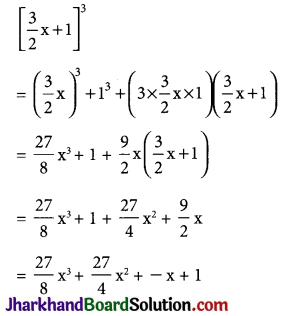(iv) $$\left[x-\frac{2}{3} y\right]^3$$
Using identity, (a – b)3 = a3 – b3 – 3ab(a – b)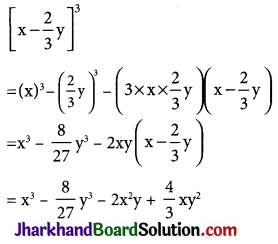Question 7.
Evaluate the following using suitable identities:
(i) (99)3
(ii) (102)3
(iii) (998)3
(i) (99)3 = (100 – 1)3
Using identity, (a – b)3 = a3 – b3 – 3ab(a – b)
(99)3 = (100 – 1)3
= (100)3 – (1)3 – (3 × 100 × 1) (100 – 1)
= 1000000 – 1 – 30000 + 300
= 970299(ii) (102)3 = (100 + 2)3
Using identity, (a – b)3 = a3 – b3 – 3ab(a – b)
(100 + 2)3
= (100)3 + (2)3 + (3 × 100 × 2) (100 + 2)
= 1000000 + 8 + 600 (100 + 2)
= 1000000 + 8 + 60000 + 1200
= 1061208

(iii) (998)3 = (1000 – 2)3
Using identity, (a – b)3 = a3 – b3 – 3ab(a – b)
= (1000)3 – (2)3 – (3 × 1000 × 2) (1000 – 2)
= 1000000000 – 8 – 6000 × (1000 – 2)
= 1000000000 – 8 – 6000000 + 12000
= 1000012000 – 6000008
= 994011992

Question 8.
Factorise each of the following:
(i) 8a3 + b3 + 12a2b + 6ab2
(ii) 8a3 – b3 – 12a2b + 6ab2
(iii) 27 – 125a3 – 135a + 225a2
(iv) 64a3 – 27b3 – 144a2b + 108ab2
(v) 27p3 – $$\frac{1}{216}-\frac{9}{2}$$p2 + $$\frac {1}{4}$$p
(i) 8a3 + b3 + 12a2b + 6ab2
Using identity, (a + b)3 = a3 + b3 + 3a2b + 3ab2
= (2a)3 + (b)3 + 3 (2a) (b) (2a + b)
= (2a + b)3
= (2a + b) (2a + b) (2a + b)

(ii) 8a3 – b3 – 12a2b + 6ab2
Using identity, (a + b)3 = a3 + b3 + 3a2b + 3ab2
= (2a)3 – (b)3 – 3 (2a)(b)(2a – b)
= (2a – b)3 = (2a – b)(2a – b)(2a – b)

(iii) 27 – 125a3 – 135a + 225a2
Using identity, (a + b)3 = a3 + b3 + 3a2b + 3ab2
= (3)3 – (5a)3 – 3(3)(5a) (3 – 5a)
= (3 – 5a)3 = (3 – 5a) (3 – 5a) (3 – 5a)

(iv) 64a3 – 27b3 – 144a2b + 108aba2
Using identity, (a + b)3 = a3 + b3 + 3a2b + 3ab2
= (4a)3 – (3b)3 – 3 (4a) (3b) (4a – 3b)
= (4a – 3b)3
= (4a – 3b) (4a – 3b) (4a – 3b)

(v) 27p3 – $$\frac{1}{216}-\frac{9}{2}$$p2 + $$\frac {1}{4}$$p
Using identity, (a + b)3 = a3 + b3 + 3a2b + 3ab2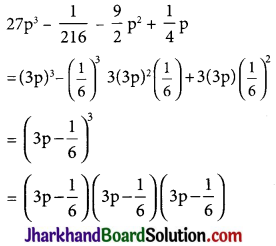Question 9.
Verify:
(i) x3 + y3 = (x + y) (x2 – xy + y2)
(ii) x3 – y3 = (x – y) (x2 + xy + y2)
(i) (x + y) (x2 – xy + y2)
We know that,
(x + y)3 = x3 + y3 + 3xy(x + y)
⇒ x3 + y3 = (x + y)3 – 3xy(x + y)
⇒ x3 + y3 = (x + y)[(x + y)2 – 3xy] {Taking (x + y) common}
⇒ x3 + y3 = (x + y) (x2 + y2 – xy)
⇒ x3 + y3 = (x + y) (x2 + xy + y2)

(ii) x3 – y3 = (x – y) (x2 + xy + y2)
We know that,
(x – y)3 = x3 + y3 – 3xy(x – y)
⇒ x3 – y3 = (x – y)3 + 3xy(x – y)
⇒ x3 + y3 = (x – y)[(x – y)2 + 3xy] {Taking (x – y) common}
⇒ x3 + y3 = (x – y) [(x2 + y2 – 2xy) + 3xy]
⇒ x3 + y3 = (x + y)(x2 + y2 + xy)

Question 10.
Factorise each of the following:
(i) 27y3 + 125z3
(ii) 64m3 – 343n3
(i) 27y3 + 125z3
Using identity, x3 + y3 = (x + y)(x2 + y2 + xy)
= (3y + 5z) [(3y)2 – 3y × 5z + (5z)2]
= (3y + 5z) [9y2 – 15yz + 25z2]

(ii) 64m3 – 343n3
Using identity, x3 + y3 = (x + y)(x2 + y2 + xy)
= (4m – 7n) [(4m)2 + 4m × 7n + (7n)2]
= (4m – 7n) [16m2 + 28mn + 49n2]

Question 11.
Factorise: 27x3 + y3 + z3 – 9xyz
27x3 + y3 + z3 – 9xyz
= (3x)3 + y3 + z3 – 3 × (3x) yz
Using identity, x3 + y3 + z3 – 3xyz
= (x + y + z)(x2 + y2 + z2 – xy – yz – xz)
27x3 + y3 + z3 – 9xyz
= (3x + y + z) [(3x)2 + (y)2 + (z)2 – 3xy – yz – 3xz]
= (3x + y + z) (9x2 + y2 + z2 – 3xy – yz – 3xz)

Question 12.
Verify that: x3 + y3 + z3 – 3xyz = $$\frac {1}{2}$$(x + y + z) [(x – y)2 + (y – z)2 + (z – x)2]
We know that,
x3 + y3 + z3 – 3xyz
= (x + y + z)(x2 + y2 + z2 – xy – yz – xz)
⇒ x3 + y3 + z3 – 3xyz
= $$\frac {1}{2}$$ × (x + y + z) 2(x2 + y2+ z2 – xy – yz – xz)
= $$\frac {1}{2}$$(x + y + z)(2x2 + 2y2 + 2z2 – 2xy – 2yz – 2xz)
= $$\frac {1}{2}$$(x + y + z) [(x2 + y2 – 2xy) + (y2 + z2 – 2yz) + (x2 + z2 – 2xz)]
= $$\frac {1}{2}$$(x + y + z) [(x – y)2+ (y – z)2 + (z – x)2]

Question 13.
If x + y + z = 0, show that x3 + y3 + z3 = 3xyz.
We know that,
x3 + y3 + z3
= (x2 + y2+ z2 – xy – yz – xz)
Now put (x + y + z) = 0,
(x2 + y2+ z2 – xy – yz – xz) – 3xyz = (0)(x2 + y2+ z2 – xy – yz – xz)
⇒ x3 + y3 + z3 = 3xyzQuestion 14.
Without actually calculating the cubes, find the value of each of the following:
(i) (-12)3 + (7)3 + (5)3
(ii) (28)3 + (-15)3 + (-13)3
(i) (-12)3 + (7)3 + (5)3
Let x = – 12, y = 7 and z = 5
We observed that,
x + y + z = -12 + 7 + 5 = 0
We know that if, x + y + z = 0,
then x3 + y3 + z3 = 3xyz
(-12)3 + (7)3 + (5)3 = 3(-12)(7)(5)
= – 1260

(ii) (28)3 + (-15)3 + (-13)3
Let x = 28, y = – 15 and z = – 13
We observed that,
x + y + z = 28 – 15 – 13 = 0
We know that if, x + y + z = 0,
then x3 + y3 + z3 = 3xyz
(28)3 + (-15)3 + (-13)3
= 3(28)(-15)(-13) = 16380

Question 15.
Give possible expressions for the length and breadth of each of the following rectangles, in which their areas are given:
(i) Area: 25a2 – 35a +12
(ii) Area: 35y2 + 13y – 12
(i) Area: 25a2 – 35a +12
Since, area is product of length and breadth therefore by factorising the given area, we can know the length and breadth of rectangle.
25a2 – 35a +12 = 25a2 – 15a – 20a + 12
= 5a(5a – 3) – 4(5a – 3) = (5a – 4) (5a -3 )
Possible expression for length = 5a – 3
Possible expression for breadth = 5a – 4

(ii) Area: 35y2 + 13y – 12
35y2 + 13y – 12 = 35y2 – 15y + 28y – 12
= 5y(7y – 3) + 4(7y – 3) = (5y + 4)(7y – 3)
Possible expression for length = (5y + 4)
Possible expression for breadth = (7y – 3)Page – 50

Question 16.
What are the possible expressions for the dimensions of the cuboids whose volumes are given below?
(i) Volume: 3x2 – 12x
(ii) Volume : 12ky2 + 8ky – 20k
(i) Volume: 3x2 – 12x
Since, volume is product of length, breadth and height therefore by factorising the given volume, we can know the length, breadth and height of the cuboid.
3x2 – 12x = 3x(x – 4)
Possible expression for length = 3
Possible expression for breadth = x
Possible expression for height = (x – 4)

(ii) Volume : 12ky2 + 8ky – 20k
Since, volume is product of length, breadth and height therefore by factorising the given volume, we can know the length, breadth and height of the cuboid.
12ky2 + 8ky – 20k = 4k(3y2 + 2y – 5) = 4k(3y2 +5y – 3y – 5)
= 4k[y(3y + 5) – 1(3y + 5)]
= 4k (3y + 5) (y – 1)
Possible expression for length = 4k
Possible expression for breadth = (3y + 5)
Possible expression for height = (y – 1)

## JAC Class 9 Maths Solutions Chapter 3 Coordinate Geometry Ex 3.1

Jharkhand Board JAC Class 9 Maths Solutions Chapter 3 Coordinate Geometry Ex 3.1 Textbook Exercise Questions and Answers.

## JAC Board Class 9th Maths Solutions Chapter 3 Coordinate Geometry Ex 3.1

Page – 54

Question 1.
How will you describe the position of a table lamp on your study table to another person?
To describe the position of a table lamp on the study table, we have to take two lines, a perpendicular and other horizontal. Considering the table as a plane and taking perpendicular line as y-axis and horizontal as x-axis. Take one corner of table as origin where both x and y axes intersect each other. Now, the length of table is y axis and breadth is x axis. From the origin, join the line to the lamp and mark a point. Calculate the distance of this point from both x and y axes and then write it in terms of coordinates.

Let the distance of point from y-axis is x and from x-axis is y then the the position of the table lamp in terms of coordinates is (x, y).Question 2.
(Street Plan): A city has two main roads which cross each other at the centre of the city. These two roads are along the North-South direction and East-West direction.

All the other streets of the city run parallel to these roads and are 200 m apart. There are 5 streets in each direction. Using 1cm = 200 m, draw a model of the city on your notebook. Represent the roads/streets by single lines. There are many cross-streets in your model. A particular cross-street is made by two streets, one running in the North – South direction and another in the East – West direction. Each cross street is referred to in the following manner: If the 2nd street running in the North – South direction and 5th in the East – West direction meet at some crossing, then we will call this cross-street (2, 5). Using this convention, find:
(i) how many cross – streets can be referred to as (4, 3).
(ii) how many cross – streets can be referred to as (3, 4)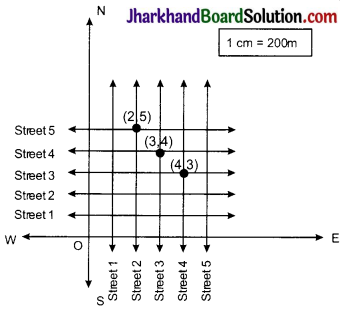(i) Only one cross-street can be referred to as (4, 3) as we see from the figure.
(ii) Only one cross-street can be referred to as (3, 4) as we see from the figure.

## JAC Class 9 Maths Solutions Chapter 2 Polynomials Ex 2.4

Jharkhand Board JAC Class 9 Maths Solutions Chapter 2 Polynomials Ex 2.4 Textbook Exercise Questions and Answers.

## JAC Board Class 9th Maths Solutions Chapter 2 Polynomials Ex 2.4

Question 1.
Determine which of the following polynomials has (x + 1) a factor:
(i) x3 + x2 + x + 1
(ii) x4 + x3 + x2 + x + 1
(iii) x4 + 3x3 + 3x2 + x + 1
(iv) x3 – x2 – (2 + $$\sqrt{2}$$)x + $$\sqrt{2}$$
(i) If (x + 1) is a factor of p(x)
=x3 + x2 + x + 1, p(-1) must be zero.
p(-1) = (-1)3 + (-1)2 + (-1) + 1
= – 1 + 1 – 1 + 1 = 0
Therefore, x + 1 is a factor of this polynomial.

(ii) If (x+ 1) is a factor of p(x)
= x4 + x3 + x2 + x + 1, p(-1) must be zero.
∴ p(-1) = (-1)4 + (-1)3 + (-1)2 + (-1) + 1
= 1 – 1 + 1 – 1 + 1 = 1 ≠ 0
Therefore, x + 1 is not a factor of this polynomial.

(iii) If (x + 1) is a factor of polynomial
x4 + 3x3 + 3x2 + x + 1, p(-1) must be 0.
P(-1) = (-1)4 + 3(-1)3 + 3(-1)2 + (-1) + 1
= 1 – 3 + 3 – 1 + 1 = 1 ≠ 0
Therefore, x + 1 is not a factor of this polynomial.

(iv) If (x + 1) is a factor of polynomial
p(x) = x3 – x2 – (2 + $$\sqrt{2}$$)x + $$\sqrt{2}$$ , p(-1) must be 0.
p(-1) = (-1)3 – (-1)2 – (2 + $$\sqrt{2}$$)(-1) + $$\sqrt{2}$$
= -1 – 1 + 2 + $$\sqrt{2}$$ + $$\sqrt{2}$$
= 2$$\sqrt{2}$$ ≠ 0
Therefore, x + 1 is not a factor of this polynomial.Question 2.
Use the Factor Theorem to determine whether g(x) is a factor of p(x) in each of the following cases:
(i) p(x) = 2x3 + x2 – 2x – 1, g(x) = x + 1
(ii) p(x) = x3 + 3x2 + 3x + 1, g(x) = x + 2
(iii) p(x) = x3 – 4x2 + x + 6, g(x) = x – 3
(i) If g(x) = x + 1 is a factor of given polynomial p(x), then p(- 1) must be zero.
P(-1) = 2(-1)3 + (-1)2 – 2(-1) – 1
= 2(-1) + 1 + 2 – 1 = 0
Hence, g(x) = x + 1 is a factor of p(x).

(ii) If g(x) = x + 2 is a factor of given polynomial p(x),then p(-2) must be 0.
p(-2) = (-2)3 + 3(-2)2 + 3(-2) + 1
= – 8 + 12 – 6 + 1 = -1 ≠ 0
Hence g(x) = x + 2 is not a factor of p(x).

(iii) If g(x) = x – 3 is a factor of given polynomial p(x), then p(3) must be 0.
p(3) = (3)3 – 4(3)2 + 3 + 6 = 27 – 36 + 9 = 0

Therefore, g(x) = x – 3 is a factor of p(x).

Page – 44

Question 3.
Find the value of k, if x – 1 is a factor of p(x) in each of the following cases:
(i) p(x) = x2 + x + k
(ii) p(x) = 2x2 + kx + $$\sqrt{2}$$
(iii) p(x) = kx2 – $$\sqrt{2}$$ x + 1
(iv) p(x) = kx2 – 3x + k
(i) If x – 1 is a factor of polynomial p(x)
= x2 + x + k, then p(1) = 0
⇒ (1)2+ 1 + k = 0
⇒ 2 + k = 0
⇒ k = – 2
Therefore, value of k is – 2.

(ii) If x – 1 is a factor of polynomial p(x) = 2x2 + kx + $$\sqrt{2}$$ , then p(1) = 0
⇒ 2(1)2 + k(1) + = 0
⇒ 2 + k + $$\sqrt{2}$$ = 0
⇒ k = – 2 – $$\sqrt{2}$$ = – (2 + $$\sqrt{2}$$)
Therefore, value of k is – (2 + $$\sqrt{2}$$ ).

(iii) If x – 1 is a factor of polynomial p(x) = kx2 – $$\sqrt{2}$$ x + 1, then p(1) = 0
⇒ k(1)2 – $$\sqrt{2}$$ (1) + 1 = 0
⇒ k – $$\sqrt{2}$$ +1 = 0
⇒ k = $$\sqrt{2}$$ – 1
Therefore, value of k is $$\sqrt{2}$$ – 1.

(iv) If x – 1 is a factor of polynomial p(x) = kx2 – 3x + k, then p(1) = 0
⇒ k(1)2 – 3(1) + k = 0
⇒ k – 3 + k= 0
⇒ 2k – 3 = 0
Therefore, value of k is $$\frac{3}{2}$$

Question 4.
Factorise:
(i) 12x2 – 7x + 1
(ii) 2x2 + 7x + 3
(iii) 6x2 + 5x – 6
(iv) 3x2 – x – 4
(i) 12x2 – 7x + 1
= 12x2 – 4x – 3x+ 1
= 4x (3x – 1) – 1 (3x – 1)
= (3x – 1) (4x – 1)

(ii) 2x2 + 7x + 3
= 2x2 + 6x + x + 3
= 2x (x + 3) + 1 (x + 3)
= (x + 3) (2x + 1)

(iii) 6x2 + 5x – 6
= 6x2 + 9x – 4x – 6
= 3x (2x + 3) – 2 (2x + 3)
= (2x + 3) (3x – 2)(iv) 3x2 – x – 4
= 3x2 – 4x + 3x – 4
= x (3x – 4) + 1 (3x – 4)
= (3x – 4) (x + 1)

Question 5.
Factorise:
(i) x3 – 2x2 – x + 2
(ii) x3 – 3x2 – 9x – 5
(iii) x3 + 13x2 + 32x + 20
(iv) 2y3 + y2 – 2y – 1
(i) Let p(x) = x3 – 2x2 – x + 2
Factors of 2 are ±1 and ± 2
By trial method, we find that p(-1) = 0
So, (x + 1) is factor of p(x)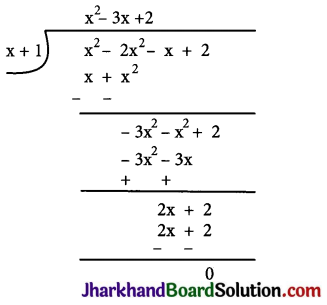Now, Dividend = Divisor × Quotient + Remainder
= (x + 1) (x2 – 3x + 2)
= (x + 1) (x2 – x – 2x + 2)
= (x+ 1) {x(x – 1) – 2(x – 1)}
= (x + 1) (x – 1) (x – 2)

(ii) Let p(x) = x3 – 3x2 – 9x – 5
Factors of 5 are ±1 and ±5
By trial method, we find that
p(5) = 0
So, (x – 5) is factor of p(x)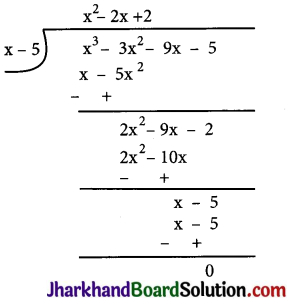Now, Dividend = Divisor × Quotient + Remainder
= (x – 5) (x2 + 2x + 1)
= (x – 5) (x2 + x + x + 1)
= (x – 5) {x(x + 1) +l(x + 1)}
= (x – 5) (x + 1) (x + 1)

(iii) Let p(x) = x3 + 13x2 + 32x + 20
Factors of 20 are ±1, ±2, ±4, ±5, ±10 and ±20
By trial method, we find that
P(-1) = 0
So, (x+1) is factor ofp(x)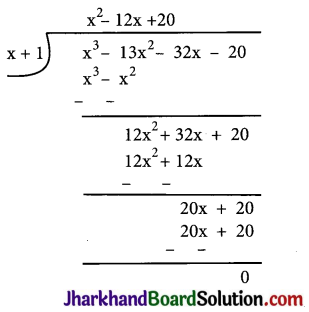Now, Dividend = Divisor × Quotient + Remainder
= (x + 1) (x2 + 12x + 20)
= (x + 1) (x2 + 2x + 10x + 20)
= (x + 1) {x(x+2) +10(x+2)}
= (x + 1) (x+2) (x+10)(iv) Let p(y) = 2y3 + y2 – 2y – 1
Factors of ab = 2 × (-1) = – 2 are ±1 and ±2
By trial method, we find that p(1) = 0
So, (y – 1) is factor of p(y)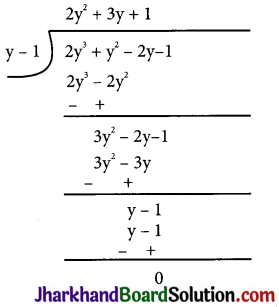Now, Dividend = Divisor × Quotient + Remainder
= (y – 1) (2y2 + 3y + 1)
= (y – 1) (2y2 + 2y + y + 1)
= (y – 1) {2y(y + 1) + 1(y + 1)}
= (y – 1 )(2y + 1) (y + 1)

## JAC Class 9 Maths Solutions Chapter 2 Polynomials Ex 2.3

Jharkhand Board JAC Class 9 Maths Solutions Chapter 2 Polynomials Ex 2.3 Textbook Exercise Questions and Answers.

## JAC Board Class 9th Maths Solutions Chapter 2 Polynomials Ex 2.3

Page – 40

Question 1.
Find the remainder when x3 + 3x2 + 3x + 1 is divided by
(i) x + 1
(ii) x – $$\frac{1}{2}$$
(iii) x
(iv) x + π
(v) 5 + 2x
(i) x + 1
Let p(x) = x3 + 3x2 + 3x + 1 be divided by x + 1
By remainder theorem, remainder = P(-1)
= (-1)3 + 3 (-1)2 + 3(-1) + 1
= -1 + 3 – 3 + 1
= 0
Therefore, the remainder is 0.

(ii) x – $$\frac{1}{2}$$
By remainder theorem,
Remainder = p($$\frac{1}{2}$$)
= ($$\frac{1}{2}$$)3 + 3($$\frac{1}{2}$$)2 + 3($$\frac{1}{2}$$) + 1
= $$\frac{1}{8}$$ + $$\frac{3}{4}$$ + $$\frac{3}{2}$$ + 1
= $$\frac{27}{8}$$
Therefore, the remainder is $$\frac{27}{8}$$.

(iii) x
By remainder theorem,
Remainder = p (0)
= 03 + 3(0)2 + 3(0) + 1
= 1
Therefore, the remainder is 1.

(iv) x + π
By remainder theorem,
Remainder = p (-π)
= (-π)3 + 3 (-π)2 + 3 (-π) + 1
= -π3 + 3π2 – 3π + 1
Therefore, the remainder is -π3 + 3π2 – 3π + 1.(v) 5 + 2x
By remainder theorem,
Remainder = p($$– \frac{5}{2}$$)
= $$\left(-\frac{5}{2}\right)^3+3\left(-\frac{5}{2}\right)^2+3\left(-\frac{5}{2}\right)+1$$
= $$\frac{-125+150-60+8}{8}$$
= $$– \frac{27}{8}$$
Therefore, the remainder is $$– \frac{27}{8}$$.

Question 2.
Find the remainder when x3 – ax2 + 6x – a is divided by x – a.
Lef f(x) = x3 – ax2 + 6x – a be divided by x – a
By remainder theorem,
Remainder = f(a)
= a3 – a(a)2 + 6(a) – a
= a3 – a3 + 6a – a
= 5a
Therefore, remainder obtained is 5a when x3 – ax2 + 6x – a is divided by x – a.

Question 3.
Check whether 7+3x is a factor of 3x3 + 7x.
Ans. Let f(x) = 3x2 + 7x be divided by 7 + 3x
As 7 + 3x = 0 gives
x = $$– \frac{7}{3}$$
By Remainder theorem,
Remainder = f($$-\frac{7}{3}$$)
= $$3\left(-\frac{7}{3}\right)^3+7\left(-\frac{7}{3}\right)$$
= $$-\frac{343}{9}-\frac{49}{3}$$
= $$\frac{-343-147}{9}$$
= $$-\frac{490}{9}$$ ≠ 0
As remainder is not zero, so 7 + 3x is not a factor of 3x3 + 7x.

## JAC Class 9 Maths Solutions Chapter 2 Polynomials Ex 2.2

Jharkhand Board JAC Class 9 Maths Solutions Chapter 2 Polynomials Ex 2.2 Textbook Exercise Questions and Answers.

## JAC Board Class 9th Maths Solutions Chapter 2 Polynomials Ex 2.2

Page – 34

Question 1.
Find the value of the polynomial 5x + 4x2 + 3 at
(i) x = 0
(ii) x = – 1
(iii) x = 2 ,
(i) p(x) = 5x + 4x2 + 3
p(0) = 5(0) + 4(0)2 + 3 = 3

(ii) p(x) = 5x + 4x2 + 3
P(-1) = 5(-1) + 4(-1)2 + 3
= -5 + 4(1) + 3 = 2

(iii) p(x) = 5x + 4x2 + 3
p(2) = 5(2) + 4(2)2 + 3
= 10 + 16 + 3 = 29

Question 2.
Find p(0), p(1) and p(2) for each of the following polynomials:
(i) p(y) = y2 – y + 1
(ii) p(x) = x3
(iii) p(t) = 2 + t + 2t2 – t3
(iv) p(x) = (x – 1) (x + 1)
(i) p(y) = y2 – y + 1
P(0) = (0)2 – (0) + 1 = 1
p(1) = (1)2 – (1) + 1 = 1
p(2) = (2)2 – (2) + 1 = 3

(ii) p(x) = x3
P(0) = (0)3 = 0
p(1) = (1)3 = 1
p(2) = (2)3 = 8

(iii) p(t) = 2 + t + 2t2 – t3
p(0) = 2 + 0 + 2 (0)2 – (0)3 = 2
P(1) = 2 + (1) + 2(1)2 – (1)3
= 2 + 1 + 2 – 1 = 4
p(2) = 2 + 2 + 2(2)2 – (2)3
= 2 + 2 + 8 – 8 = 4

(iv) p(x) = (x – 1) (x + 1)
P(0) = (0 – 1) (0 + 1) = (-1) (1) = – 1
p(1) = (1 – 1) (1 + 1) = 0(2) = 0
p(2) = (2 – 1) (2 + 1) = 1(3) = 3Page – 35

Question 3.
Verify whether the following are zeroes of the polynomial, indicated against them.
(i) p(x) = 3x + 1, x = $$– \frac{1}{3}$$
(ii) p(x) = 5x – π, x = $$\frac{4}{5}$$
(iii) p(x) = x2 – 1, x = 1, – 1
(iv) p(x) = (x + 1) (x – 2), x = – 1, 2
(v) p(x) = x2, x = 0
(vi) p(x) = lx + m, x = $$– \frac{m}{l}$$
(vii) p(x) = 3x2 – 1, x = $$-\frac{1}{\sqrt{3}}, \frac{2}{\sqrt{3}}$$
(viii) p(x) = 2x + 1, x = $$\frac{1}{2}$$
(i) If x = $$– \frac{1}{3}$$ is a zero of polynomial p(x) then p($$– \frac{1}{3}$$) = 0
p($$– \frac{1}{3}$$) = 3($$– \frac{1}{3}$$) + 1 = -1 + 1 = 0
Therefore, x = $$– \frac{1}{3}$$ is a zero of polynomial p(x) = 3x + 1.

(ii) If x = $$\frac{4}{5}$$ is a zero of polynomial
p(x) = 5x – π then p($$\frac{4}{5}$$) should be 0.
p($$\frac{4}{5}$$) = 5($$\frac{4}{5}$$) – π = 4 – π ≠ 0
Therefore, x = $$\frac{4}{5}$$ is not a zero of given polynomial p(x) = 5x – π.

(iii) If x = 1 and x = – 1 are zeroes of polynomial p(x) = x2 – 1, then p(1) and p(-1) each should be 0.
p(1) = (1)2 – 1 = 0 and
P(-1) = (-1)2 – 1 = 0
Hence, x = 1 and – 1 are zeroes of the polynomial p(x) = x2 – 1.

(iv) If x = -1 and x = 2 are zeroes of polynomial p(x) = (x + 1) (x – 2), then p( -1) and p(2) each should be 0.
p(-1) = (-1 + 1) (-1 – 2) = 0 (-3) = 0, and
p(2) = (2 + 1) (2 – 2) = 3 (0) = 0
Therefore, x = – 1 and x = 2 are zeroes of the polynomial p(x) = (x+1) (x – 2).(v) If x = 0 is a zero of polynomial p(x) = x2, then p(0) should be zero.
Here, p(0) = (0)2 = 0
Hence, x = 0 is a zero of the polynomial p(x) = x2.

(vi) If x = $$– \frac{m}{l}$$ is a zero of polynomial p(x) = lx + m, then p($$– \frac{m}{l}$$)= 0 should be 0.
p($$– \frac{m}{l}$$) = l($$– \frac{m}{l}$$) + m = -m + m = 0
Therefore x = ($$– \frac{m}{l}$$) is a zero of polynomial p(x) = lx + m

(vii) If x = $$-\frac{1}{\sqrt{3}}$$ and x = $$\frac{2}{\sqrt{3}}$$ are zeros of polynomial p(x) = 3x2 – 1, then p($$-\frac{1}{\sqrt{3}}$$) and p($$\frac{2}{\sqrt{3}}$$)should be 0.
p($$\frac{-1}{\sqrt{3}}$$) = $$3\left(\frac{-1}{\sqrt{3}}\right)^2$$ – 1 = 3($$\frac{1}{3}$$)-1 = 1 – 1 = 0
p($$\frac{2}{\sqrt{3}}$$) = $$3\left(\frac{2}{\sqrt{3}}\right)^2$$ – 1 = 3($$\frac{4}{3}$$) – 1 = 4 – 1 = 3 ≠ 0
Therefore, x = $$\frac{-1}{\sqrt{3}}$$ is a zero of polynomial p(x) = 3x2 – 1 but, x = $$\frac{2}{\sqrt{3}}$$ is not a zero of this polynomial.

(viii) If x = $$\frac{1}{2}$$ is a zero of polynomial p(x) = 2x + 1then p($$\frac{1}{2}$$) should be 0.
p($$\frac{1}{2}$$) = 2($$\frac{1}{2}$$) + 1 = 1 + 1 = 2 ≠ 0
Therefore, x = $$\frac{1}{2}$$ is not a zero of given polynomial p(x) = 2x + 1

Question 4.
Find the zero of the polynomial in each of the following cases:
(i) p(x) = x + 5
(ii) p(x) = x – 5
(iii) p(x) = 2x + 5
(iv) p(x) = 3x – 2
(v) p(x) = 3x
(vi) p(x) = ax, a ≠ 0
(vii) p(x) = cx + d, c ≠ 0, d, are real numbers.
(i) p(x) = x + 5
p(x) = 0
x + 5 = 0
x = – 5
Therefore, x = – 5 is a zero of polynomial p(x) = x + 5 .

(ii) p(x) = x – 5
p(x) =0
x – 5 = 0
x = 5
Therefore, x = 5 is a zero of polynomial p(x) = x – 5.(iii) p(x) = 2x + 5
p(x) = 0
2x + 5 = 0
2x = – 5
x = $$– \frac{5}{2}$$
Therefore, x = $$– \frac{5}{2}$$ is a zero of polynomial p(x) = 2x + 5.

(iv) p(x) = 3x – 2
p(x) = 0
3x – 2 = 0
x = $$\frac{2}{3}$$
Therefore, x = $$\frac{2}{3}$$ is a zero of polynomial p(x) = 3x – 2.

(v) p(x) = 3x
p(x) = 0
3x = 0
x = 0
Therefore, x = 0 is a zero of polynomial p(x) = 3x.

(vi) p(x) = ax, a ≠ 0
p(x) = 0
ax = 0
x = 0
Therefore, x = 0 is a zero of polynomial p(x) = ax.

(vii) p(x) = cx + d, c ≠ 0
p(x) = 0
cx + d = 0
x = $$– \frac{d}{c}$$
Therefore, x = $$– \frac{d}{c}$$ is a zero of polynomial p(x) = cx + d.

## JAC Class 9 Maths Solutions Chapter 2 Polynomials Ex 2.1

Jharkhand Board JAC Class 9 Maths Solutions Chapter 2 Polynomials Ex 2.1 Textbook Exercise Questions and Answers.

## JAC Board Class 9th Maths Solutions Chapter 2 Polynomials Ex 2.1

Question 1.
Which of the following expressions are polynomials in one variable and which are not? State reasons for your answer.
(i) 4x2 – 3x + 7
(ii) y2 + $$\sqrt{2}$$
(iii) 3$$\sqrt{t}$$ + t$$\sqrt{2}$$
(iv) y + $$\frac{2}{y}$$
(v) x10 + y3 + t50
(i) 4x2 – 3x + 7
There is only one variable x with whole number powers so this is a polynomial in one variable.

(ii) y2 + $$\sqrt{2}$$
There is only one variable y with whole number powers so this is a polynomial in one variable.

(iii) 3$$\sqrt{t}$$ + t$$\sqrt{2}$$
There is only one variable t but in 3$$\sqrt{t}$$ power of t is $$\frac{1}{2}$$ which is not a whole number. So, 3$$\sqrt{t}$$ + t$$\sqrt{2}$$ is not a polynomial.

(iv) y + $$\frac{2}{y}$$
There is only one variable y but $$\frac{2}{y}$$ = 2y-1 So, the power of y is not a whole number in the second term. Hence, y + $$\frac{2}{y}$$ is not a polynomial.

(v) x10 + y3 + t50
There are three variables x, y and t. So, this is not a polynomial in one variable.

Question 2.
Write the coefficients of x2 in each of the following:
(i) 2 + x2 + x
(ii) 2 – x2 + x3
(iii) $$\frac{π}{2}$$x2 + x
(iv) $$\sqrt{2}$$ – 1
(i) Coefficient of x2 = 1
(ii) Coefficient of x2 = -1
(iii) Coefficient of x2 = $$\frac{π}{2}$$
(iv) Coefficient of x2 = 0Question 3.
Give one example each of a binomial of degree 35, and of a monomial of degree 100.
3x35 + 7 and 4x100 are binomials of degree 35 and monomial of degree 100 respectively.

Question 4.
Write the degree of each of the following polynomials:
(i) 5x3 + 4x2 + 7x
(ii) 4 – y2
(iii) 5t – $$\sqrt{7}$$
(iv) 3
(i) 5x3 has highest power in the given polynomial, which is 3. Therefore, degree of polynomial is 3.
(ii) -y2 has highest power in the given polynomial, which is 2. Therefore, degree of polynomial is 2.
(iii) 5t has highest power in the given polynomial, which is 1. Therefore, degree of polynomial is 1.
(iv) There is no variable in the given polynomial. Therefore, degree of polynomial is 0.

Question 5.
Classify the following as linear, quadratic and cubic polynomials:
(i) x2 + x
(ii) x – x3
(iii) y + y2 + 4
(iv) 1 + x
(v) 3t
(vi) r2
(vii) 7x3
(i) x2 + x Quadratic Polynomial
(ii) x – x3 Cubic Polynomial
(iii) y + y2 + 4 Quadratic Polynomial
(iv) 1 + x Linear Polynomial
(v) 3t Linear Polynomial
(vi) r2 Quadratic Polynomial
(vii) 7x3 Cubic Polynomial

## JAC Class 9 Maths Solutions Chapter 1 Number Systems Ex 1.6

Jharkhand Board JAC Class 9 Maths Solutions Chapter 1 Number Systems Ex 1.6 Textbook Exercise Questions and Answers.

## JAC Board Class 9th Maths Solutions Chapter 1 Number Systems Exercise 1.6

Question 1.
Find:
(i) $$64^{\frac{1}{2}}$$
(ii) $$32^{\frac{1}{5}}$$
(iii) $$125^{\frac{1}{3}}$$
(i) $$64^{\frac{1}{2}}=\left(2^6\right)^{\frac{1}{2}}=2^{6 \times \frac{1}{2}}=2^3=8$$
(ii) $$32^{\frac{1}{5}}=\left(2^5\right)^{\frac{1}{5}}=2^{5 \times \frac{1}{5}}=2$$
(iii) $$125^{\frac{1}{3}}=\left(5^3\right)^{\frac{1}{3}}=5^{3 \times \frac{1}{3}}=5$$

Question 2.
Find:
(i) $$9^{\frac{3}{2}}$$
(ii) $$32^{\frac{2}{5}}$$
(iii) $$16^{\frac{3}{4}}$$
(iv) $$125^{\frac{-1}{3}}$$
(i) $$9^{\frac{3}{2}}=\left(3^2\right)^{\frac{3}{2}}=3^3=27$$
(ii) $$32^{\frac{2}{5}}=\left(2^5\right)^{\frac{2}{5}}=2^2=4$$
(iii) $$16^{\frac{3}{4}}=\left(2^4\right)^{\frac{3}{4}}=2^3=8$$
(iv) $$125^{\frac{-1}{3}}=\frac{1}{(125)^{\frac{1}{3}}}=\frac{1}{\left(5^3\right)^{\frac{1}{3}}}=\frac{1}{5}$$Question 3.
(i) $$2^{\frac{2}{3}} \cdot 2^{\frac{1}{5}}$$
(ii) $$\left(\frac{1}{3^3}\right)^7$$
(iii) $$\frac{11^{\frac{1}{2}}}{11^{\frac{1}{4}}}$$
(iv) $$7^{\frac{1}{2}} \cdot 8^{\frac{1}{2}}$$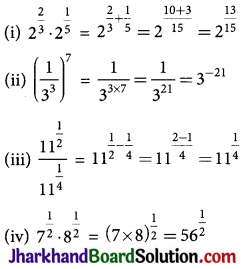## JAC Class 9 Maths Solutions Chapter 1 Number Systems Ex 1.5

Jharkhand Board JAC Class 9 Maths Solutions Chapter 1 Number Systems Ex 1.5 Textbook Exercise Questions and Answers.

## JAC Board Class 9th Maths Solutions Chapter 1 Number Systems Exercise 1.5

Question 1.
Classify the following numbers as rational or irrational:
(i) 2 – $$\sqrt{5}$$
(ii) (3 + $$\sqrt{23}$$) – $$\sqrt{23}$$
(iii) $$\frac{2 \sqrt{7}}{7 \sqrt{7}}$$
(iv) $$\frac{1}{\sqrt{2}}$$
(v) 2π
(i) 2 – $$\sqrt{5}$$ = 2 – 2.2360679…
= -0.2360679…
Since the number is non-terminating non-recurring therefore, it is an irrational number.

(ii) (3 + $$\sqrt{23}$$) – $$\sqrt{23}$$
3 + $$\sqrt{23}$$ – $$\sqrt{23}$$
= 3 = $$\frac{3}{1}$$
The number is rational number as it can represented in $$\frac{p}{q}$$ form, where p, q ∈ Z, q ≠ 0

(iii) $$\frac{2 \sqrt{7}}{7 \sqrt{7}}$$
The number is rational number as it can represented in $$\frac{p}{q}$$ form, where p, q ∈ Z, q ≠ 0

(iv) $$\frac{1}{\sqrt{2}}$$
= 0.7071067811…
Since the number is non-terminating non-recurring therefore, it is an irrational number.

(v) 2π = 2 × 3.1415… = 6.2830…
Since the number is non-terminating non-recurring therefore, it is an irrational number.

Question 2.
Simplify each of the following expressions:
(i) (3 + $$\sqrt{3}$$) (2 + $$\sqrt{2}$$)
(ii) (3 + $$\sqrt{3}$$) (3 – $$\sqrt{3}$$)
(iii) ($$\sqrt{5}$$ + $$\sqrt{2}$$)2
(iv) ($$\sqrt{5}$$ – $$\sqrt{2}$$)($$\sqrt{5}$$ + $$\sqrt{2}$$)
(i) (3 + $$\sqrt{3}$$) (2 + $$\sqrt{2}$$)
= 3 × 2 + 2$$\sqrt{3}$$ + 3$$\sqrt{2}$$ + $$\sqrt{3}$$ ×$$\sqrt{2}$$
= 6 + 2$$\sqrt{3}$$ + 3$$\sqrt{2}$$ + $$\sqrt{6}$$

(ii) (3 + $$\sqrt{3}$$) (3 – $$\sqrt{3}$$)
= 32– ($$\sqrt{3}$$)2 = 9 – 3 = 6
[∵ (a + b) (a – b) = a2 – b2]

(iii) ($$\sqrt{5}$$ + $$\sqrt{2}$$)2
= ($$\sqrt{5}$$)2 + ($$\sqrt{2}$$)2 + 2 × $$\sqrt{5}$$ × $$\sqrt{2}$$
[∵ (a + b)2 = a2 + b2 + 2ab]
= 5 + 2 + 2 × $$\sqrt{5}$$ ×$$\sqrt{2}$$
= 7 + 2$$\sqrt{10}$$

(iv) ($$\sqrt{5}$$ – $$\sqrt{2}$$)($$\sqrt{5}$$ + $$\sqrt{2}$$)
= ($$\sqrt{5}$$)2 – ($$\sqrt{2}$$)2
= 5 – 2 = 3
[∵ (a + b) (a – b) = a2 – b2]Question 3.
Recall, 7t is defined as the ratio of the circumference (say c) of a circle to its diameter (say d). That is, n = c/d. This seems to contradict the fact that n is irrational. How will you resolve this contradiction?
There is no contradiction. When we measure a value with a scale, we only obtain an approximate value. We never obtain an exact value. Therefore, we may not realise that either c or d is irrational. The value of n is almost equal to 22/7 or 3.14159…

Question 4.
Represent $$\sqrt{9.3}$$ on the number line.
Step 1: Draw a line segment AB of 9.3 units. Extend it to C so that BC is of 1 unit.
Step 2: Now, AC = 10.3 units. Find the midpoint of AC and name it as O.
Step 3: Draw a semicircle with radius OC and centre O.
Step 4: Draw a perpendicular line segment BD to AC at point B which intersects the semicircle at D. Also, Join OD.
Step 5: Now, OBD is a right angled triangle.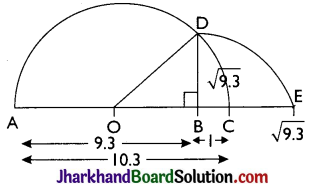Here, OD = 10.3/2 (radius of semicircle),
OC = $$\frac{10.3}{2}$$, BC = 1
OB = OC – BC = ($$\frac{10.3}{2}$$) – 1 = $$\frac{8.3}{2}$$
Using Pythagoras theorem
OD2 = BD2 + OB2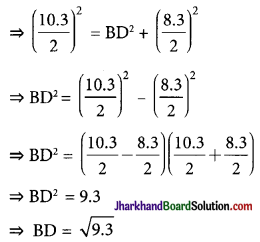Thus, the length of BD is $$\sqrt{9.3}$$ units.

Step 6: Taking BD as radius and B as centre draw an arc which touches the line segment AC extended at the point E. The point E is at a distance of $$\sqrt{9.3}$$ from B as shown in the figure.Question 5.
Rationalise the denominators of the following:
(i) $$\frac{1}{\sqrt{7}}$$
(ii) $$\frac{1}{\sqrt{7}-\sqrt{6}}$$
(iii) $$\frac{1}{\sqrt{5}+\sqrt{2}}$$
(iv) $$\frac{1}{\sqrt{7}-2}$$
(i) $$\frac{1}{\sqrt{7}}$$ = $$\frac{1 \times \sqrt{7}}{\sqrt{7} \times \sqrt{7}}$$ = $$\frac{\sqrt{7}}{7}$$
(ii) $$\frac{1}{\sqrt{7}-\sqrt{6}}$$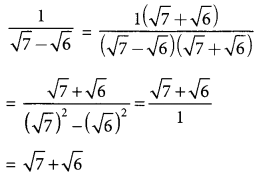(iii) $$\frac{1}{\sqrt{5}+\sqrt{2}}$$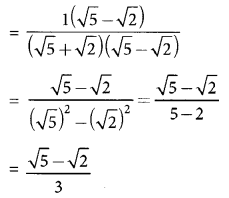(iv) $$\frac{1}{\sqrt{7}-2}$$